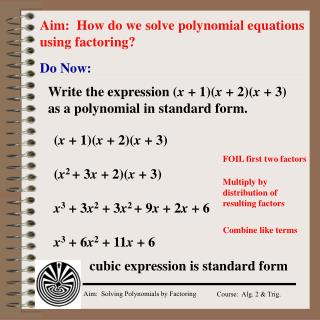DownloadDownload PresentationAim: How do we solve polynomial equations using factoring?

# Aim: How do we solve polynomial equations using factoring?

Download Presentation## Aim: How do we solve polynomial equations using factoring?

- - - - - - - - - - - - - - - - - - - - - - - - - - - E N D - - - - - - - - - - - - - - - - - - - - - - - - - - -
##### Presentation Transcript

1. Aim: How do we solve polynomial equations using factoring? Do Now: Write the expression (x + 1)(x + 2)(x + 3) as a polynomial in standard form. (x + 1)(x + 2)(x + 3) FOIL first two factors (x2 + 3x + 2)(x + 3) Multiply by distribution of resulting factors x3 + 3x2 + 3x2 + 9x + 2x + 6 Combine like terms x3 + 6x2 + 11x + 6 cubic expression is standard form

2. Solving Polynomials by Factoring Factor: 2x3 + 10x2 + 12x GCF 2x(x2 + 5x + 6x) Factor trinomial 2x(x+ 3)(x + 2) Solve: 2x3 + 10x2 + 12x = 0 2x(x2 + 5x + 6x) = 0 2x(x+ 3)(x + 2) = 0 2x = 0 (x+ 3) = 0 (x + 2) = 0 Set factors equal to zero (Zero Product Property) x = 0, -2, -3

3. Graphical Solutions Solve: 2x3 + 10x2 + 12x = 0 2x(x+ 3)(x + 2) = 0 x = 0, -2, -3 Cubic equation in Standard form ax3 + bx2 + cx + d = y y-intercept

4. Model Problem Find the zeros of y = (x – 2)(x + 1)(x + 3) Zeros/roots/x-intercepts are found at y = 0 (x-axis) 0 = (x – 2)(x + 1)(x + 3) (x – 2) = 0 (x + 1) = 0 (x + 3) = 0 Set factors equal to zero x = -3, -1, 2 y-intercept? (-2) (1) (3) = -6 is the product of last terms of binomial factors y = x3 + 2x2 – 5x – 6

5. -1 Synthetic Division & Factors Divide x3 – x2 + 2 by x + 1 using synthetic division Since there is no remainder x + 1 is a factor of x3 – x2 + 2 1 -1 0 2 2 -1 -2 0 1 2 -2 x2 – 2x + 2 0 quotient remainder Remainder Theorem (x2 – 2x + 2)(x + 1) = x3 – x2 + 2 Solve: x3 – x2 + 2 = 0 (x2 – 2x + 2)(x + 1) = 0 (x2 – 2x + 2) = 0 (x + 1) = 0 Set factors equal to zero Quadratic Formula x = -1 x = 1 ± i

6. Synthetic Division, Factors and Graphing Solve: x3 – x2 + 2 = 0 (x2 – 2x + 2)(x + 1) = 0 (x2 – 2x + 2) = 0 (x + 1) = 0 Set factors equal to zero Quadratic Formula x = -1 x = 1 ± i

7. Model Problem -3 Use synthetic division to show that x + 3 is a factor of y = 2x3 + 11x2 + 18x + 9 then solve 2x3 + 11x2 + 18x + 9 = 0 2 11 18 9 -15 -6 -9 0 2 3 5 2x3 + 11x2 + 18x + 9 = 0 2x2 + 5x + 3 (2x2 + 5x + 3)(x + 3) = 0 (2x + 3)(x + 1)(x + 3) = 0 (2x + 3) = 0 (x + 1) = 0 (x + 3) = 0 x = (-3, -3/2, -1)

8. Factor by Grouping x3 – 2x2 – 3x + 6 (x3 – 2x2) – (3x – 6) Group terms Factor Groups x2(x – 2) – 3(x – 2) Distributive Property (x2 - 3)(x – 2)

9. Model Problem Factor: 4x3 – 6x2 + 10x – 15 (4x3 – 6x2) + (10x – 15) Group terms Factor Groups 2x2(2x – 3) + 5(2x – 3) Distributive Property (2x2 + 5)(2x – 3) Factor: x3 – 2x2 – 4x + 8 (x3 – 2x2) – (4x – 8) x2(x – 2) + 4(x – 2) (x2 + 4)(x – 2)

10. (x2 – 3) = 0  x = Model Problem Solve: x3 – 3x2 – 3x + 9 = 0 Group (x3 – 3x2) – (3x – 9) = 0 x2(x – 3) – 3(x – 3) = 0 Factor (x2 – 3)(x – 3) = 0 (x– 3) = 0  x = 3

11. u2 – 3u + 2 = 0 Solving Quadratic Form Equations x4 – 3x2 + 2 = 0 u = x2 u2 – 3u + 2 = 0 Quadratic form (u– 1)(u – 2) = 0 Factor (x2 – 1)(x2 – 2) = 0 Substitute (x– 1)(x + 1)(x2 – 2) = 0 Factor (x– 1) = 0  x = 1 Set factors = 0 & solve for x (x+ 1) = 0  x = -1 (x2+ 2) = 0  x =

12. Model Problem Solve: x4 – x2 = 12